Numerical simulation of Helmholtz coil

HOME / Applications / Numerical simulation of Helmholtz coil
WEBINAR
Simultaneous Wireless Information and Power Transfer
Thursday, January 27, 2022
Time
SESSION 1
SESSION 2
CET (GMT +1)
03:00 PM
08:00 PM
EST (GMT -4)
09:00 AM
02:00 PM
Used Tools:Helmholtz coil

Helmholtz coil is a device for producing a region of nearly uniform magnetic field, named after the German physicist Hermann von Helmholtz. It consists of two solenoid electromagnets, as shown in Figure 1, on the same axis. Besides creating magnetic fields, Helmholtz coils are also used in scientific apparatus to cancel external magnetic fields, such as the Earth's magnetic field.
A Helmholtz pair consists of two identical circular magnetic coils (solenoids) that are placed symmetrically along a common axis, one on each side of the experimental area, and separated by a distance h equal to the radius R of the coil. Each coil carries an equal electric current in the same direction.

Magnetic field has significant impact on plants and seeds both at the physical and molecular level. The Helmholtz coil that can generate a uniform magnetic field in a large area can be ideal for Biomagnetic experiments, and this was the main motive of the Helmholtz coil design, it had bioelectric perspective. Bioelectric experiments with plants and seeds require large volume of uniform magnetic field for exposure. The ordinary Helmholtz coil, 30 cm diameter, has insignificant volume of uniform magnetic field for biomagnetic experiments which requires large number of samples. The Helmholtz coil was designed for treating 200 seeds at a time. The uniform magnetic field is generated at center of the structure and covers approximately 1/3 the total volume. It was designed such that it is driven by both AC and DC current source. The Helmholtz coil was also designed such that it would withstand temperature rise even when driven for long periods of time. This is necessary because bioelectric experiments involve long period of exposure in the magnetic field, sometimes requiring several days of exposure.

The equation for the field strength at the center of the coils is: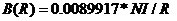B=magnetic field, in gauss
N=number of turns of wire on each coil
I=current through each turn of wire, in amperes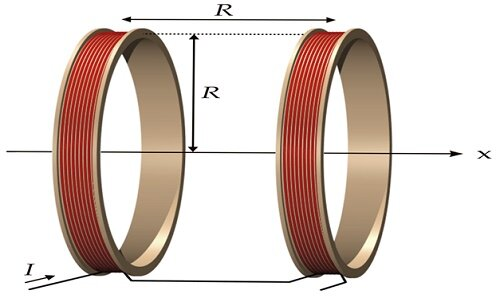Figure 1 – model of Helmholtz coil

The 3D model shown in Figure 2 was built in SolidWorks CAD.  Table 1 contains the Helmholtz coil parameters and dimension.

Table 1 – Parameters and dimensions of Helmholtz coil

 Diameter (cm) Coil diameter 47.5 Coil width 100 Coil depth 53 Coil height 130 Number of turns 100 Gauge 10Figure 2 - 3D model of Helmholtz coil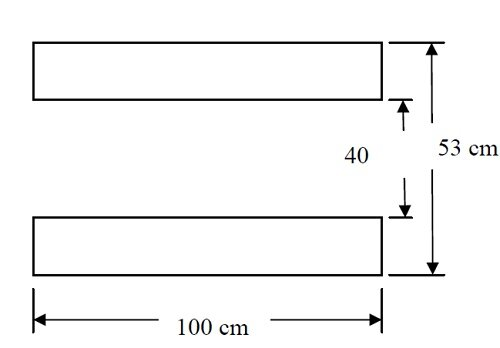Figure 3- top view of the coil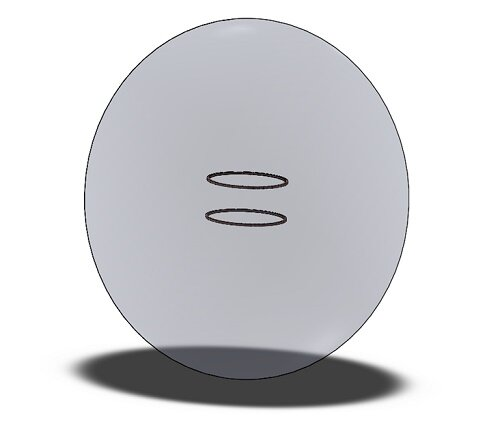Figure 4- 3D model: an air region enclosing the coil of Helmholtz

Due to the symmetry conditions only quarter of the whole model was simulated. This approach reduces the time taken to solve without compromising the solution accuracy.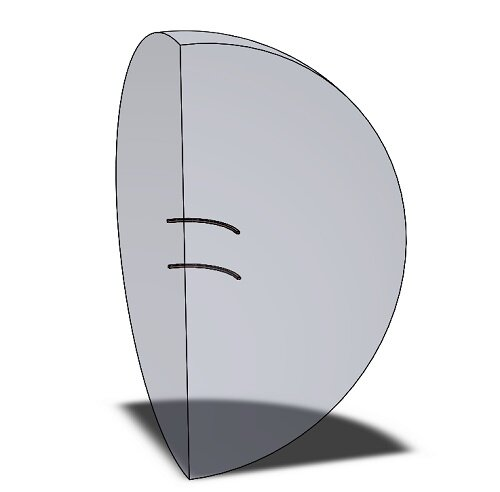Figure 5- simulated model

Numerical Simulation and Results using EMS

AC simulation

The AC Magnetic or the so-called time harmonic magnetic analysis belongs to the low-frequency electromagnetic domain or regime; i.e. displacement currents are neglected. In addition, the fields are time harmonic.

The AC Magnetic Analysis calculates the effects of alternating currents (AC) in electromagnetic devices. These effects include: Eddy currents, skin effects, power loss due to eddy currents.

The AC Magnetic solver computes the electric fields excited by a Sinusoidal (or alternating) varying voltage or a Sinusoidal (or alternating) varying current.

In this study two coils (Helmholtz coil) are defined as per Table 2.

Table 2 – AC Coils information

 Coil AC current Phase shift (degree) Coil 1 7.3 A Peak value 0 Coil 2 7.3 A Peak value 0

A tangential flux must be applied on faces of model where we want to use symmetry conditions.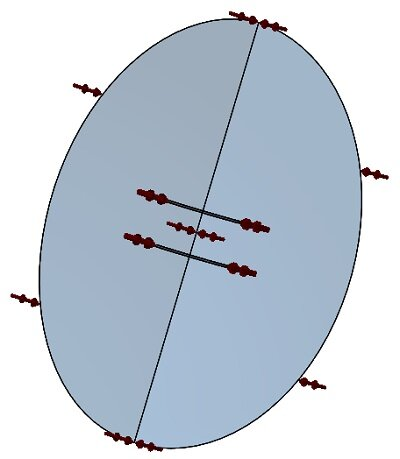Figure 6- faces where applied tangential flux

Meshing

Figure 7 shows the mesh on Helmholtz coil. Meshing is a crucial step in any FEA simulation. EMS estimates a global element size for the model taking into consideration its volume, surface area, and other geometric details. The size of the generated mesh (number of nodes and elements) depends on the geometry and dimensions of the model, element size, mesh tolerance, and mesh control. In the early stages of design analysis where approximate results may suffice, you can specify a larger element size for a faster solution. For a more accurate solution, a smaller element size may be required.

We can control the mesh size from one body to another depending on its dimensions and its importance in the result by applying a mesh control. Applied mesh controls for this model are listed in table 4.

Table 4 - Mesh controls applied for this model

 Name Mesh Size (mm) Bodies/ components Mesh control 1 5.00 Coils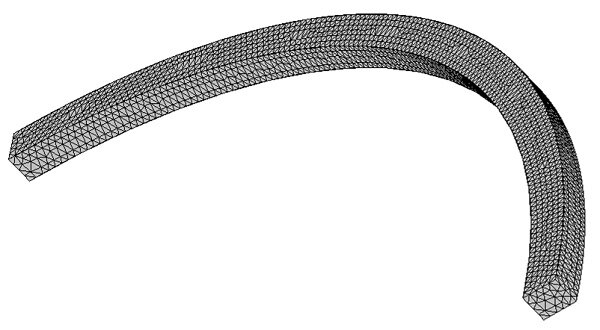Figure 7- Meshed coil

ElectroMagnetic results

As mentioned before AC Magnetic solver in EMS allows to compute flux density B, field intensity H, applied and total current density (applied + induced) etc. Also, a results table is generated containing all circuit parameters (inductance, resistance, losses etc).

Figure 8 shows flux density Bz along the axis of two coils. The results are similar to what is published in reference  page 55. As per the property of Helmholtz coil, the flux density is uniform along the axis of coils which is proved by numerical simulation and experimental results .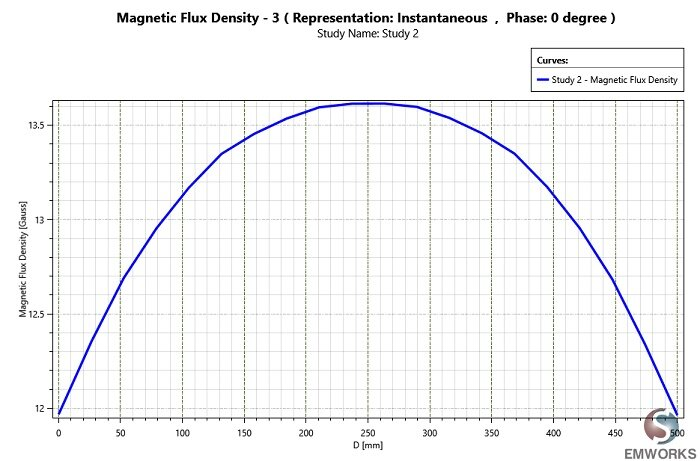Figure 8- Bz  along z axis

DC simulation

In addition to AC, Helmholtz coil can be driven by DC source, so a magnetostic simulation is needed in this case.
Magnetostatic or the so-called DC Magnetic Field analysis belongs to the low-frequency electromagnetic domain or regime; i.e. displacement currents are neglected. In addition, the fields depend on position only. They do not depend on time. Furthermore, the size of the object is much smaller than the wavelength.

The Magnetostatic Analysis, linear and non-linear, calculates the magnetic fields produced by one the following:

• A Permanent magnet.
• A Steady DC electric current.

In the same tree, create new study (Magnetostatic study).

Table 5 - DC coils information

 Coil DC current Coil 1 4.8 A Coil 2 4.8 A

ElectroMagnetic results

Flux density, magnetic field intensity, applied current and force density can be generated by Magnetostatic study. Also, it allows to compute the circuit parameters.

Figure 11 shows the Bz along z-axis of Helmholtz coil which conform with results in . The flux density is uniform around the center of coil.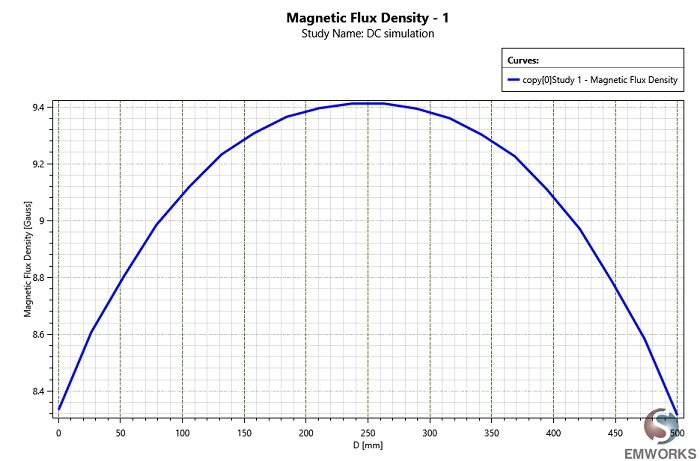Figure 9 - Bz along z axis with DC current

Conclusion

Electromagnetic simulation using EMS can help engineers to design and size their Helmholtz coil for any application. By using both the AC and DC simulation, all real life conditions of the Helmholtz coil can be simulated.

References

:DESIGN AND CONSTRUCTION OF HELMHOLTZ COIL FOR BIOMAGNETIC STUDIES ON SOYBEAN “by Dr SHASHI RAJ GYAWALI , the Faculty of the Graduate School at the University of Missouri-Columbia